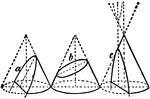### Cone

Sections of a cone. a, parabola; b, ellipse; c, hyperbola.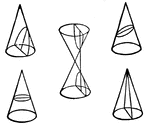### Conic Sections

Conic sections, cones divided by a plane.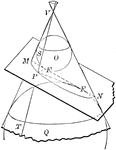### Cone depicting Conic Sections

Diagram of a cone with spheres and cut by a plane to depict the conic sections.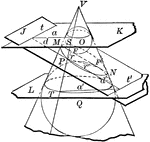### Cone depicting Conic Sections

Diagram of a cone with inscribed spheres and cut by various planes to depict the conic sections: circle,…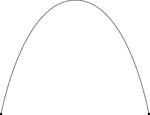### Line, Curved

A curved line with endpoints. This illustration resembles a parabola.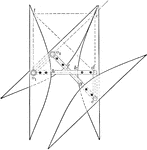### Rolling of Equal Hyperbolas

Illustration of the rolling of equal hyperbolas. If two equal hyperbolas are placed so that the distances…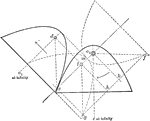### Rolling of Equal Parabolas

Illustration of the rolling of equal parabolas. "The two parabolas may be considered as two ellipses…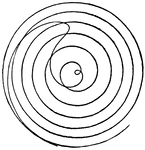### Fermat's Spiral

Also called a parabolic spiral, it is a type of Archimedean Spiral. A spiral is defined as "a plane…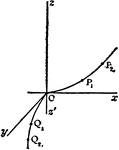### Hyperbolic Parabaloid

Three dimensional representation of a variable hyperbola moving parallel to itself along the parabolas…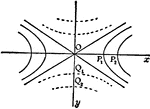### Hyperbolic Parabaloid

Two dimensional representation of variable hyperbola moving parallel to itself along the parabolas as…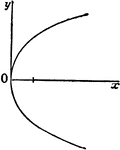### Parabola

One of the three species of conic sections is the parabola.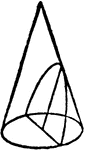### Parabola

Illustration showing a parabola as a curve formed by the intersection of the surface of a cone with…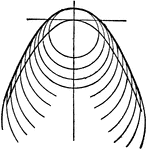### Parabola

Illustration showing a parabola with curve lines.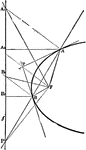### Parabola Foci Properties

The segments between the point of intersection of two tangents to a conic and their points of contact…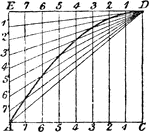### Construction of Parabola Given Base and Height

Illustration used to draw a parabola when given the base and height.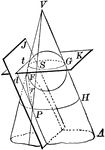### Cone Intersected by a Plane to Form a Parabola

Diagram of a cone intersected by plane J to form a parabola. Also pictured is a circle formed by the…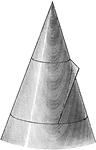### Conic Section Showing Parabola

Illustration showing the definition of a parabola as a conic section. "The section of a right circular…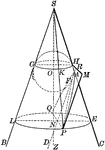### Conic Section Showing Parabola

Illustration showing the definition of a parabola as a conic section. "The section of a right circular…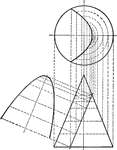### Conic Section Showing Parabola

Illustration showing the definition of a parabola as a conic section. The plane cuts the cone parallel…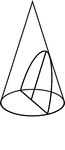### Conic Section Showing A Parabola

Illustration showing the definition of a parabola as a conic section. The section of a right circular…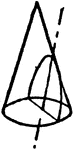### Conic Section Showing A Parabola

An illustration showing the intersection of a plane and a cone. The cone is intersected by a plane parallel…### Construction Of A Parabola

An illustration showing how to construct a parabola by plotting. "Having given the axis, vertex, and…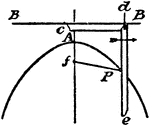### Construction Of A Parabola

An illustration showing how to construct a parabola using a pencil and a string. "Having given the two…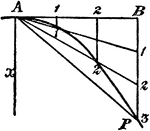### Construction Of A Parabola

An illustration showing how to construct a parabola. "Given the vertex A, axis x, and a point P. Draw…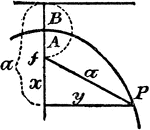### Construction Of A Parabola

An illustration showing how to construct a parabola. "Given the axis of ordinate B, and vertex A. Take…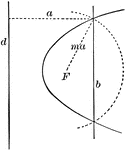### Construction of a Parabola

Diagram showing how to construct a parabola when given the directrix (d), the focus (F) and the eccentricity…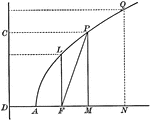### Parabola With Coordinates and Latus Rectum

Illustration of a parabola showing that any point of a parabola is the mean proportional between the…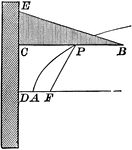### Parabola With Focus and Directrix

Illustration of a parabola - a curve which is the locus of a point that moves in a plane so that its…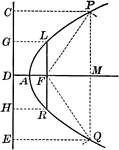### Parabola With Focus and Directrix

Illustration of a parabola - a curve which is the locus of a point that moves in a plane so that its…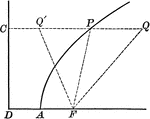### Point on Parabola

Illustration of a parabola showing point P is equidistant to the focus and the directrix.### Point on Parabola

Illustration of a parabola. "If a line PT is drawn from any point P of the curve, bisecting the angle…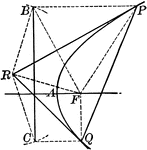### Tangent to a Parabola

Illustration of a tangent line drawn from an external point to a parabola.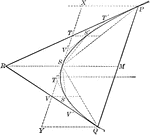### Tangents and Chords of a Parabola

Illustration showing that the area of a parabolic segment made by a chord is two thirds the area of…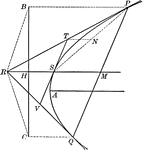### Tangents to a Parabola

Illustration showing that if two tangents RP and RQ are drawn from a point R to a parabola, the line…